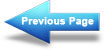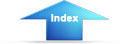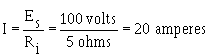voltage is caused by the INTERNAL RESISTANCE of the battery (the opposition to current offered by the electrolyte in the battery). All sources of electromotive force have some form of internal resistance which causes a drop in terminal voltage as current flows through the source."> Source resistanceCustom SearchShort circuitMatter, Energy, and ElectricityPower transfer and efficiencySOURCE RESISTANCE
A meter connected across the terminals of a good 1.5-volt battery reads about 1.5 volts. When the same battery is inserted into a complete circuit, the meter reading decreases to something less than 1.5 volts. This difference in terminal voltage is caused by the INTERNAL RESISTANCE of the battery (the opposition to current offered by the electrolyte in the battery). All sources of electromotive force have some form of internal resistance which causes a drop in terminal voltage as current flows through the source.

This principle is illustrated in figure 3-35, where the internal resistance of a battery is shown as Ri. In the schematic, the internal resistance is indicated by an additional resistor in series with the battery. The battery, with its internal resistance, is enclosed within the dotted lines of the schematic diagram. With the switch open, the voltage across the battery terminals reads 15 volts. When the switch is closed, current flow causes voltage drops around the circuit. The circuit current of 2 amperes causes a voltage drop of 2 volts across Ri . The 1-ohm internal battery resistance thereby drops the battery terminal voltage to 13 volts. Internal resistance cannot be measured directly with a meter. An attempt to do this would damage the meter.

Figure 3-35. - Effect of internal resistance.The effect of the source resistance on the power output of a dc source may be shown by an analysis of the circuit in figure 3-36. When the variable load resistor (RL) is set at the zero-ohm position (equivalent to a short circuit), current (I) is calculated using the following formula:This is the maximum current that may be drawn from the source. The terminal voltage across the short circuit is zero volts and all the voltage is across the resistance within the source.

Figure 3-36. - Effect of source resistance on power output.If the load resistance (RL) were increased (the internal resistance remaining the same), the current drawn from the source would decrease. Consequently, the voltage drop across the internal resistance would decrease. At the same time, the terminal voltage applied across the load would increase and approach a maximum as the current approaches zero amps.Integrated Publishing, Inc. - A (SDVOSB) Service Disabled Veteran Owned Small Business